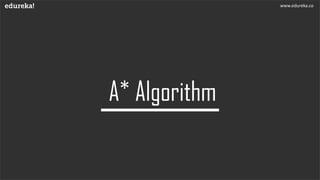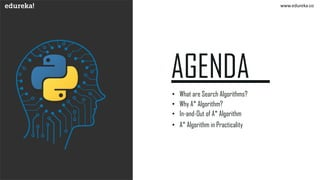Ce diaporama a bien été signalé.
Le téléchargement de votre SlideShare est en cours. ×

# A star algorithm | A* Algorithm in Artificial Intelligence | Edureka

Publicité
Publicité
Publicité
Publicité
Publicité
Publicité
Publicité
Publicité
Publicité
Publicité
PublicitéChargement dans…3
×

## Consultez-les par la suite

1 sur 25 Publicité

# A star algorithm | A* Algorithm in Artificial Intelligence | Edureka

** Artificial Intelligence and Deep Learning: https://www.edureka.co/ai-deep-learni... **
This Edureka PPT on 'A Star Algorithm' teaches you all about the A star Algorithm, the uses, advantages and disadvantages and much more. It also shows you how the algorithm can be implemented practically and has a comparison between the Dijkstra and itself.

Check out our playlist for more videos: http://bit.ly/2taym8X

Follow us to never miss an update in the future.
Instagram: https://www.instagram.com/edureka_learning/
Castbox: https://castbox.fm/networks/505?country=in

** Artificial Intelligence and Deep Learning: https://www.edureka.co/ai-deep-learni... **
This Edureka PPT on 'A Star Algorithm' teaches you all about the A star Algorithm, the uses, advantages and disadvantages and much more. It also shows you how the algorithm can be implemented practically and has a comparison between the Dijkstra and itself.

Check out our playlist for more videos: http://bit.ly/2taym8X

Follow us to never miss an update in the future.
Instagram: https://www.instagram.com/edureka_learning/
Castbox: https://castbox.fm/networks/505?country=in

Publicité
Publicité

### A star algorithm | A* Algorithm in Artificial Intelligence | Edureka

1. 1. www.edureka.co
2. 2. www.edureka.co
3. 3. www.edureka.co • What are Search Algorithms? • Why A* Algorithm? • In-and-Out of A* Algorithm • A* Algorithm in Practicality
4. 4. www.edureka.co
5. 5. What are Search Algorithms? • Moving from one place to another is a task we do almost everyday • Finding the shortest path by ourselves was difficult • We now have algorithms that help us find that shortest route www.edureka.co
6. 6. www.edureka.co
7. 7. Why A* Algorithm? Before the why, let’s understand what is A* A* is an extended BFS Algorithm that prioritizes the shortest routes that it can reach first and then the other routes. It is optimal and complete. www.edureka.co
8. 8. OP Why A* Algorithm? A* is sure to give you the best solution that is available for the problem Optimal www.edureka.co
9. 9. OP Why A* Algorithm? A* is sure to give you the best solution that is available for the problem Optimal Complete A* is to find all the possible solutions that exist for the problem www.edureka.co
10. 10. OP Why A* Algorithm? Dijkstra does not know when to stop as it does not know which is the best path and tends to compute inefficiently A* computes the best possible way and stops when the best route is found. This makes the computations effective and efficient www.edureka.co
11. 11. www.edureka.co
12. 12. In-and-Out of A* Algorithm • This formula is the heart and soul of this algorithm • These help in optimizing and finding the efficient path www.edureka.co
13. 13. In-and-Out of A* Algorithm • This parameter is used to find the least cost from one node to the other F = G + H • Responsible to find the optimal path between source and destination www.edureka.co
14. 14. In-and-Out of A* Algorithm • This parameter is the cost of moving from node to another F = G + H • The parameter changes for every movement from one node to other www.edureka.co
15. 15. In-and-Out of A* Algorithm • This parameter is the heuristic path between current node to destination F = G + H • Not actual cost but is the assumption cost from the node to destination www.edureka.co
16. 16. In-and-Out of A* Algorithm • Let’s understand how the calculation works out • Our graph here which has a source S, Destination E and other nodes S BA E 1 2 13 5 www.edureka.co
17. 17. In-and-Out of A* Algorithm The cost to enter the source is the travel cost, zero and heuristic cost which is 5 S BA E 1 2 13 5 F = G + H For source, f = g + h = 0 + 5 = 5 www.edureka.co
18. 18. In-and-Out of A* Algorithm The travel cost through A is 5 and B is 7, so we shall choose A for now S BA E 1 2 13 5 For S-A, 1 + 4 = 5 For S-B, 2 + 5 = 7 Choose S-A www.edureka.co
19. 19. In-and-Out of A* Algorithm The travel cost through SA to E is 14 and SB to E is just 7. So we choose 7 as our shortest path. S BA E 1 2 13 5 For S-A-E, (1+13) + 0 = 14 For S-B-E, (2+5) + 0 = 7 Choose S-B-E www.edureka.co
20. 20. In-and-Out of A* Algorithm S BA E 1 2 13 5 The Final Path is S-B-E www.edureka.co
21. 21. In-and-Out of A* Algorithm Algorithm A*(): Add start node to list For all the neighboring nodes, find the least cost F node Switch to the closed list For 8 nodes adjacent to the current node If the node is not reachable, ignore it. Else If the node is not on the open list, move it to the open list and calculate f, g, h. If the node is on the open list, check if the path it offers is less than the current path and change to it if it does so. Stop working when You find the destination You cannot find the destination going through all possible points www.edureka.co
22. 22. www.edureka.co
23. 23. Let’s code! www.edureka.co